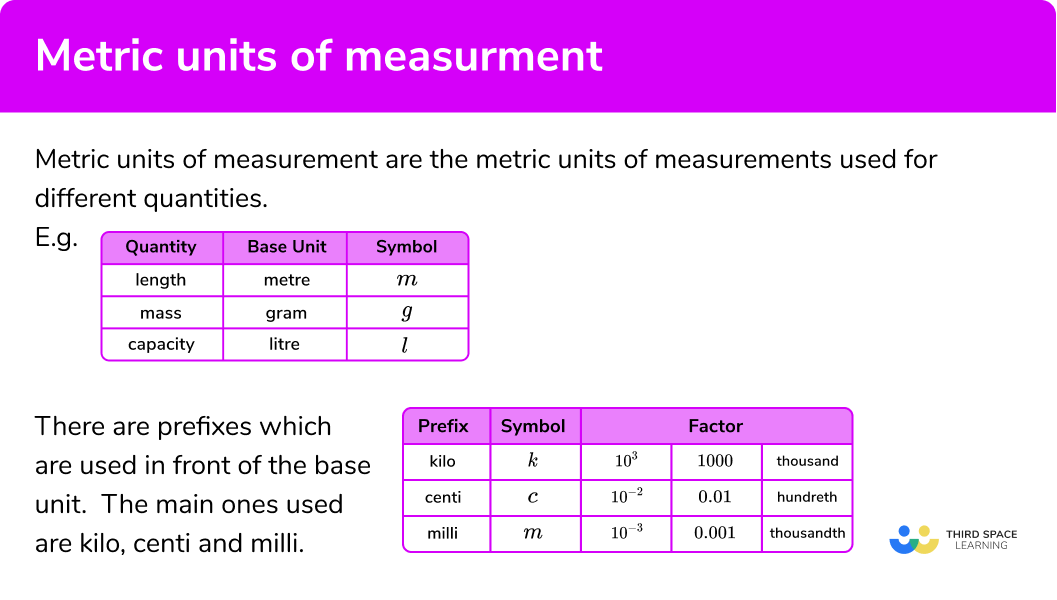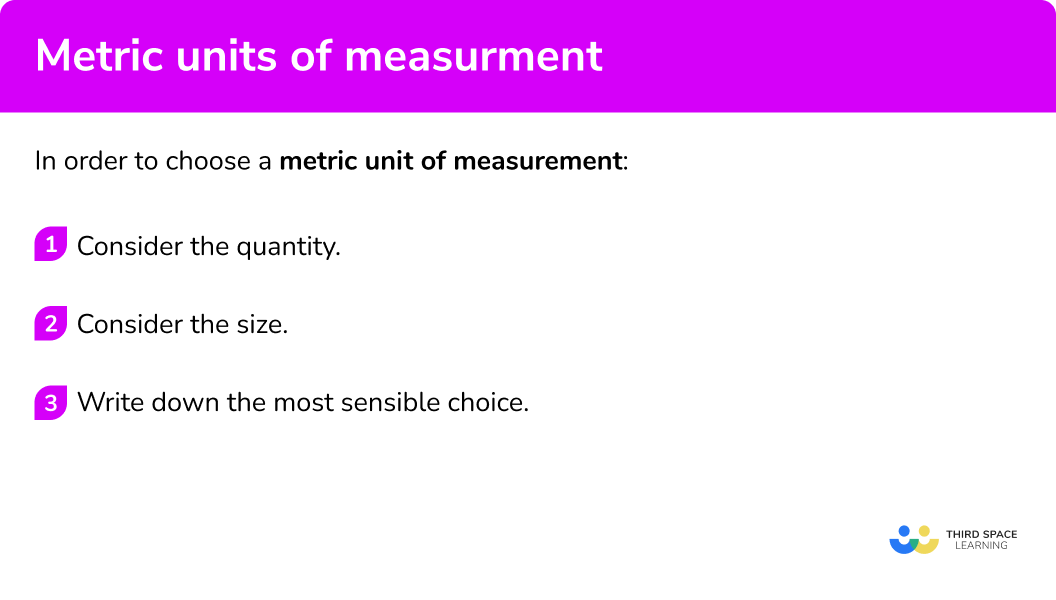# Metric Units Of Measurement

Here we will learn about metric units of measurement. We will look at common units of measurement in the metric system of measurement and how they are used.

There are also metric units of measurement worksheets based on Edexcel, AQA and OCR exam questions, along with further guidance on where to go next if you’re still stuck.

## What are metric units of measurement?

Metric units of measurement are the metric units of measurements used for different quantities.

The metric system of units of measurement is based on powers of 10.

Prefixes are used to indicate which power of 10 is involved.

The prefix “centi” means \frac{1}{100} th

E.g.

A centimetre is \frac{1}{100} th of a metre.

A centimetre is \frac{1}{100} th of a litre

The prefix “milli means \frac{1}{1000} th

E.g.

A millimetre is \frac{1}{1000} th of a metre.

A millilitre is \frac{1}{1000} th of a litre.

The prefix “kilo” which means 1000 times larger.

E.g.

A kilometre is 1000 times larger than a metre.

E.g.

The metric unit of measurement for length is the metre (m) ,

The metric unit of measurement for mass is the gram (g) ,

The metric unit of measurement for capacity is the litre (l) .

For smaller quantities or larger quantities we can adapt these units of measurement using prefixes which are used in front of the base unit.

The main ones used are kilo, centi and milli.

### What are metric units of measurement?### The unit of length

For distances between towns we can use kilometres (km) .

For the height of a tree we can use a metre (m)

For the width of a book we can use centimetres (cm) .

For small items such as a paperclip we can use the smaller unit of measurement, millimetres (mm) .

### The unit of mass

To weigh people we use kilograms (kg) .

To weigh single pieces of fruit or vegetables we would use grams (g)

For the weight of a car we could use a less common unit of mass which is the tonne (t). \; 1 tonne is 1000 \ kg .

### The unit of capacity (or unit of volume)

For the capacity of a bath, or a pond or a bucket we would use litres (l) .

For smaller items such as a cup we would use the smaller unit millilitres (ml)

Sometimes bottles and cans of drink use a less common unit of capacity.

## How to choose a metric unit of measurement

In order to choose a metric unit of measurement:

1. Consider the quantity.
2. Consider the size.
3. Write down the most sensible choice.

### How to choose a metric unit of measurement## Related lessons on units of measurement

Metric units of measurement is part of our series of lessons to support revision on units of measurement. You may find it helpful to start with the main units of measurement lesson for a summary of what to expect, or use the step by step guides below for further detail on individual topics. Other lessons in this series include:

## Metric units of measurements examples

### Example 1: units of length

Write down a sensible metric unit of measurement for:

The width of a calculator.

1. Consider the quantity.

We need a length, so we need to consider the measurement units based on the metre.

2Consider the size.

A calculator could be measured by a 15 \ cm or 30 \ cm ruler. So, centimetres might be the best metric unit of measurement.

3Write down the most sensible choice.

Centimetres is the most sensible unit of measurement for the width of a calculator.

### Example 2: units of length

Write down a sensible metric unit of measurement for:

The distance between London and Paris.

We need a length, so we need to consider the measurement units based on the metre.

The distance between two cities is far. So, kilometres might be the best metric unit of measurement.

Kilometres is the most sensible unit of measurement for the distance between London and Paris.

### Example 3: units of mass

Write down a sensible metric unit of measurement for:

The mass of a cow

We need a mass, so we need to consider either grams or kilograms.

A cow is large, so we can choose the larger unit.

Kilograms is the most sensible unit of measurement for the mass of a cow.

### Example 4: units of length

Write down a sensible metric unit of measurement for:

The mass of a pencil.

We need a mass, so we need to consider either grams or kilograms.

A pencil is small, so we can choose the smaller unit.

Grams is the most sensible unit of measurement for the mass of a pencil.

### Example 5: units of capacity (volume)

Write down a sensible metric unit of measurement for:

The volume of a tea cup.

We need a capacity, so we need to consider either litres or millilitres

A teacup is small, so we can choose the smaller unit.

Millilitres is the most sensible unit of measurement for the capacity of a tea cup.

### Example 6: units of capacity (volume)

Write down a sensible metric unit of measurement for:

The volume of a pond.

We need a capacity, so we need to consider either litres or millilitres

A pond is large, so we can choose the larger unit.

Litres is the most sensible unit of measurement for the capacity of a pond.

### Common misconceptions

• Metric or imperial units

A common error is to confuse metric units with Imperial units of measurement. Imperial units are an older system of measurements but some are still used in everyday life.

E.g.

1 \ inch≈2.5 \ cm

1 \ foot≈30 \ cm

1 \ mile≈1.5 \ km

### Practice units of measurement questions

1. Choose the most sensible metric unit to measure:

The height of a building

metreskilogramsinchesmillimetresWe need a unit for length – so kilograms can be ruled out.  We need a metric unit – so inches can be ruled out.  A building is large, so metres are the best unit.

2. Choose the most sensible metric unit to measure:

The weight of a tins of beans

kilogramsouncesmillilitresgramsWe need a unit for mass – so millilitres can be ruled out.  We need a metric unit – so ounces can be ruled out.  The weight of a tin of beans is small, so grams are the best unit.

3. Choose the most sensible metric unit to measure:

The thickness of an exercise book

metresmillimetresyardsmillilitresWe need a unit for length – so millilitres can be ruled out.  We need a metric unit – so yards can be ruled out.  The thickness of an exercise book is very small, so millimetres are the best unit.

4. Choose the most sensible metric unit to measure:

The volume of a teaspoon

kilogramspintsmillilitreslitresWe need a unit for capacity – so kilograms can be ruled out.  We need a metric unit – so pints can be ruled out.  The volume of a teaspoon is very small, so millilitres are the best unit.

5. Choose the most sensible metric unit to measure:

The weight of a sheep

gramskilogramsstonescentimetresWe need a unit for mass – so centimetres can be ruled out.  We need a metric unit – so stones can be ruled out.  The weight of a sheep is large, so kilograms are the best unit.

6. Choose the most sensible metric unit to measure:

The volume of a lake

kilometreslitresmillilitresgallonsWe need a unit for capacity – so kilometres can be ruled out.  We need a metric unit – so gallons can be ruled out.  The volume of a lake is very large, so litres are the best unit.

### Metric units of measurement GCSE questions

1.  Circle the best metric unit of measurement for:

The weight of a mouse

centimetres      kilograms      ounces      grams

(1 marks)

grams

(1)

2.   Circle the best metric unit of measurement for:

The volume of a swimming pool

gallons      litres      kilograms      millilitres

(1 marks)

litres

(1)

3.  Write down a sensible metric unit of measurement for:

(a)  The weight of a person

(b)  The width of a table

(c)  The volume of water in a glass

(3 marks)

kilograms

(1)

centimetres

(1)

millilitres

(1)

## Learning checklist

You have now learned how to:

• Choose the most sensible metric units for measuring different quantities

## Still stuck?

Prepare your KS4 students for maths GCSEs success with Third Space Learning. Weekly online one to one GCSE maths revision lessons delivered by expert maths tutors.

Find out more about our GCSE maths tuition programme.